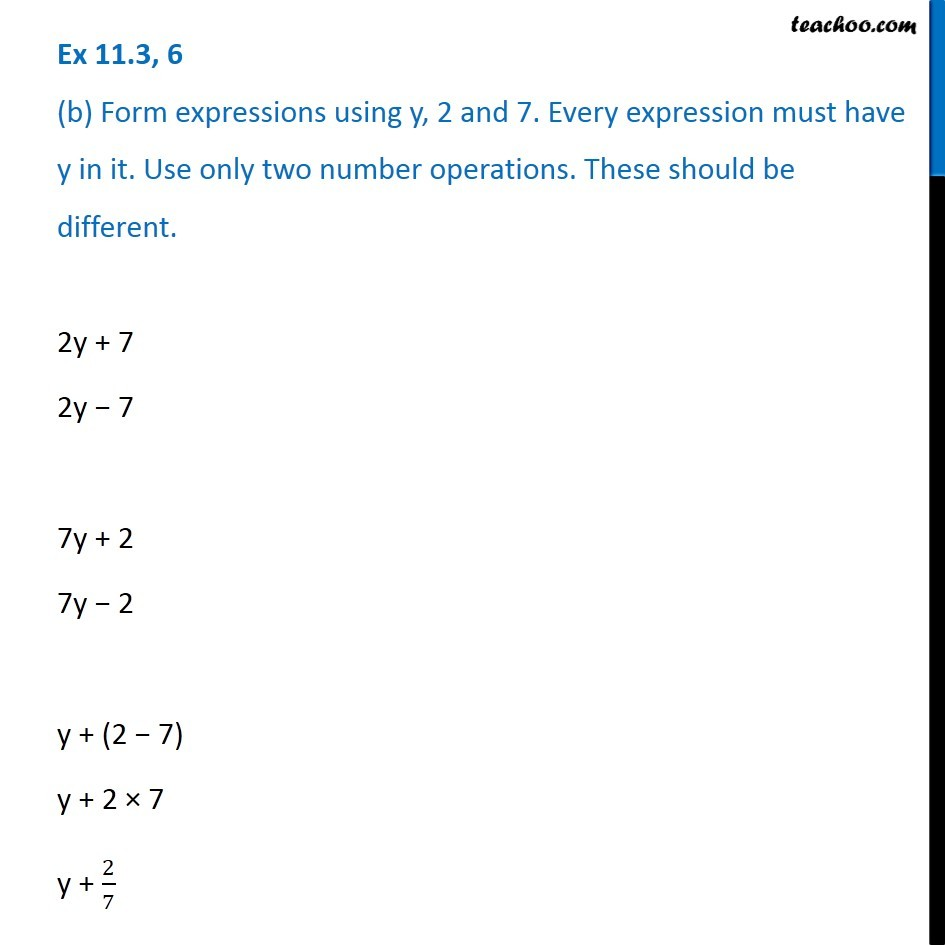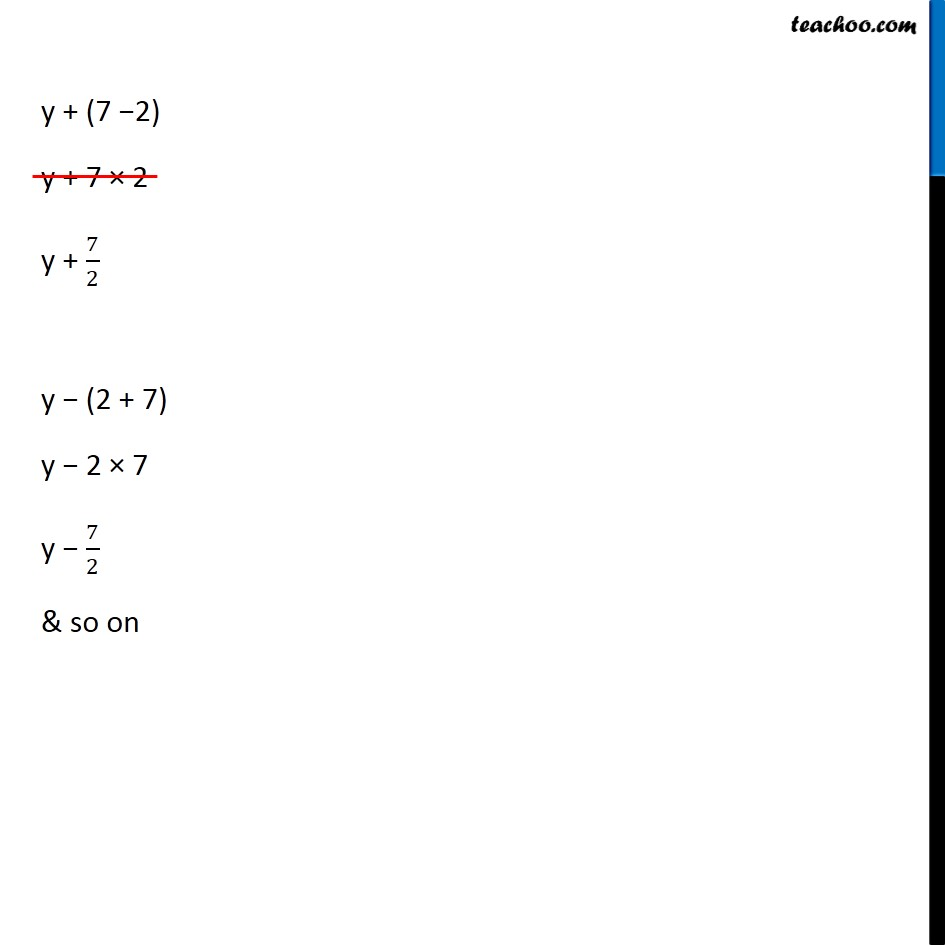Creating Basic Algebra Expressions - WorksheetLearn in your speed, with individual attention - Teachoo Maths 1-on-1 Class

### Transcript

Question 6 (b) Form expressions using y, 2 and 7. Every expression must have y in it. Use only two number operations. These should be different.2y + 7 2y − 7 7y + 2 7y − 2 y + (2 − 7) y + 2 × 7 y + 2/7 y + (7 −2) y + 7 × 2 y + 7/2 y − (2 + 7) y − 2 × 7 y − 7/2 & so on